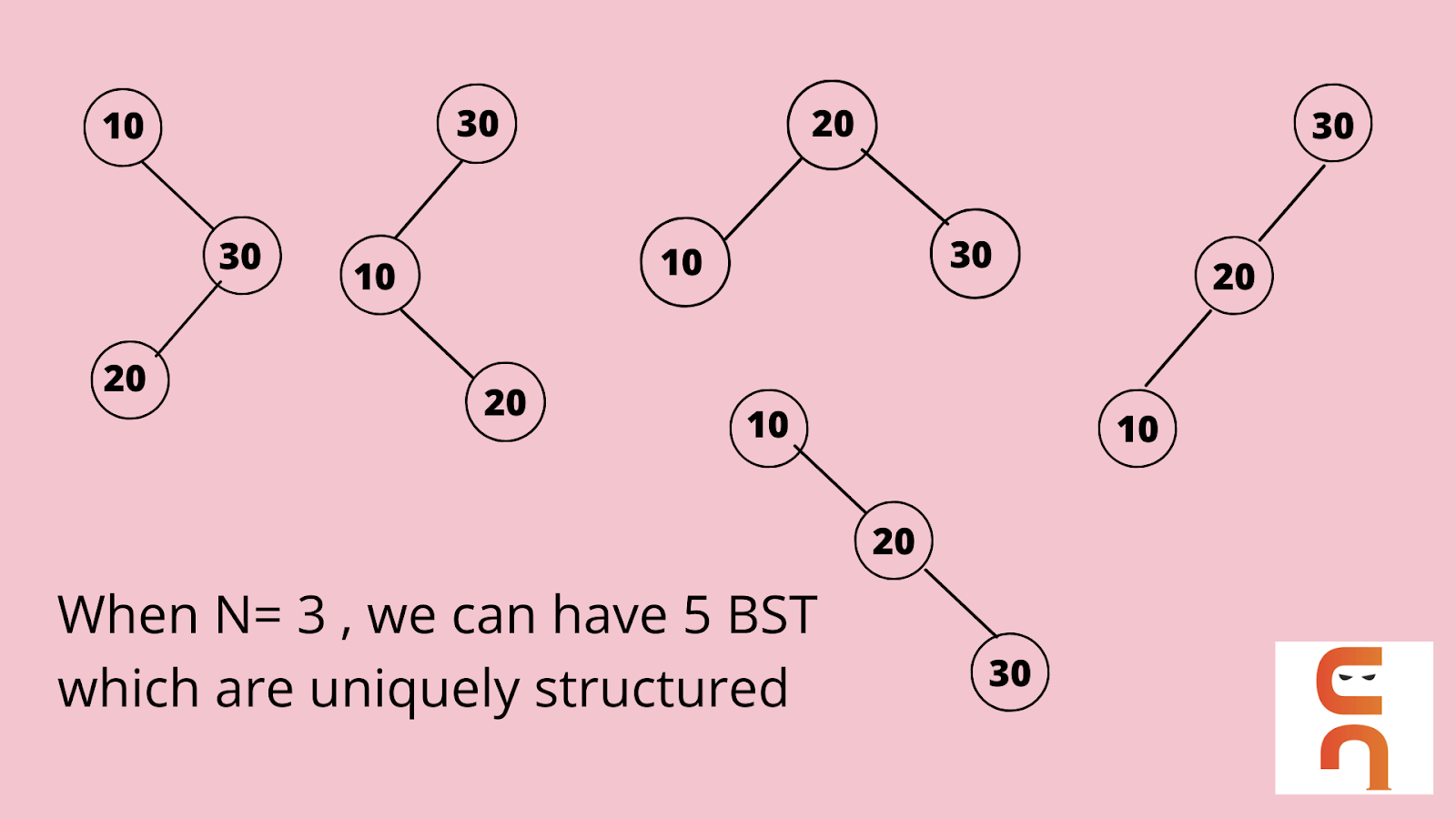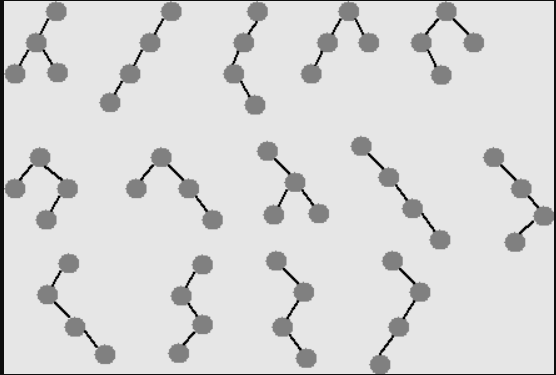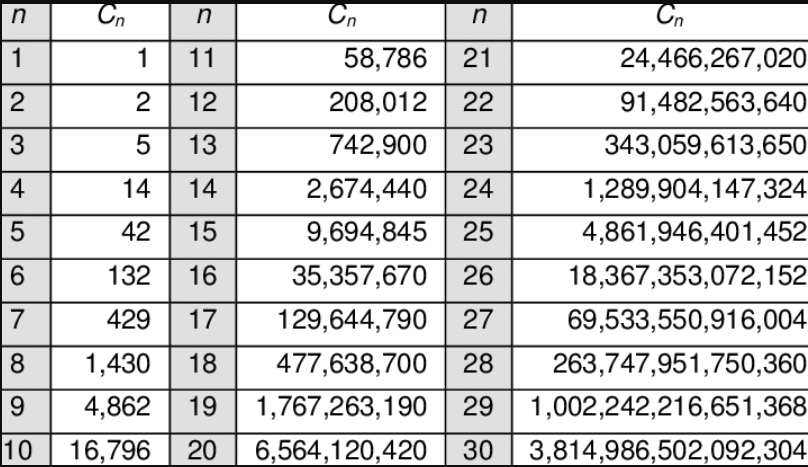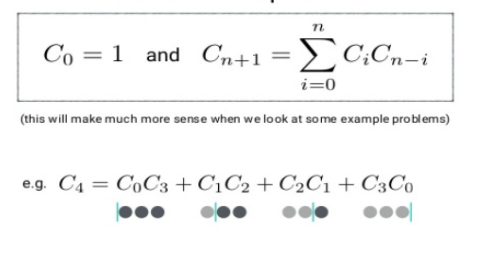# Unique Binary Search Trees

## Introduction

This article will cover the problem in which we are given a number ‘N,’ and we need to print the count of uniquely structured binary search trees that can be created having nodes from 1 to N.

For example, Let’s assume that we are given N = 3, and for the sake of the example, let’s take the nodes as 10, 20, and 30.

We can have uniquely structured binary search trees using the above-given information.Observe the above example. We had N=3 which means the number of nodes given to us was 3, and we found that there can be five structurally unique Binary Search Trees we can construct using just three nodes. Similarly, if we have two nodes, the number of unique binary trees we could construct is two. Let’s go through the problem Statement to find the desired output.

## Problem Statement

We are given ‘N’, that is the number of nodes, and we are asked to return the count of all the structurally unique Binary Trees generated, which store values from 1 to ‘N’.

The input from the user will be ‘N’ that is the number of nodes in the tree.

The output should be a count of all the uniquely structured binary search trees containing values from 1 to N.

There are four approaches to this problem.

1. Recursive Approach
2. Recursive Approach using Memoization
3. Bottom-Up (Dynamic Programming)
4. Catalan Number Approach

In this article, we will be discussing all these approaches with their Algorithms and Implementation in Java.Before understanding the four approaches, remember that a Binary Search tree is a rooted binary tree, which stores all the lesser values than the root node in the left subtree and the greater values than the root node in the right subtree, with left and right subtrees also being binary search trees. To find a uniquely structured binary search tree, we need to count a tree in which the structure of a binary search tree is not the same as a previously counted binary search tree.

Let’s start discussing the different approaches and their algorithms.

## Recursion

In the above example, we saw that we got five distinct unique binary search trees when we had three nodes.

What would happen if we had four nodes?

Let’s see using an example,If we consider nodes 10, 20, 30, and 40,

1. When we take 10 as a root node, the values {20, 30, and 40} will be the right subtree of the root. Since we have 3 values as the right subtree, those can be mapped in a tree to form 5 unique binary search trees.

2. When we take 20 as the root node there will be one left child and we know that using 1 node we can form only 1 unique tree. Over at the right side of the node, we have values {30,40} using these two values we can form two unique binary search trees. To count the final we multiply the (1 X 2) - we get 2 unique binary search trees.

3. Similarly, when we take 30 as the root node, we have {10,20} on the left subtree that would result in two unique binary search trees,  and the right subtree has only one value that is 40, which could get us a unique binary search tree. Therefore, even in this, we get two unique binary search trees because of the product of the left and right subtrees.

4. When we consider {40} as a root node, we get three nodes at the left subtree and 0 nodes on the right subtree, this makes the outcome of total binary search trees as five. Because we have already seen in the above example that using 3 nodes we get 5 unique binary search trees.

We can have the above 14 (which is the sum of 5+2+2+5) unique binary search trees with just four nodes. This is because we will construct trees considering each node as a root.

Let numTrees(n) be the total number of unique BSTs with n nodes. The total number of BSTs with root node as i is given as numTrees(i-1) * numTrees(n-i) as the arrangement of nodes in left and right subtrees are independent. Hence summing over every root node i, the summation formula we can derive using the above explanation is:

numTrees(n) = 1n numTrees(i-1)* numTrees(n-i), where numTrees(0) = 1 and numTrees(1) = 1

Let’s understand the algorithm for the approach,

We will use the concept of Recursion to find the count of unique binary search trees in this approach.

### Algorithm

The above algorithm checks for all the conditions and returns the total possible unique binary search trees that are likely using the given number of nodes.

This algorithm is a brute force approach for this problem, as it does display the desired result but takes exponential time. The base condition checks if the node is just 0 or 1, this means only one tree is possible for these kinds of trees.  In the else block, we set a count variable to track the count number of trees possible.

### Implementation in Java

``````class CodingNinjas {
static long numTrees(int n) {
if(n==0|| n==1)
return 1;
else {
long count =0;
for(int i=1;i<=n;i++) {
count+= numTrees(i-1)*numTrees(n-i);
}
return count;
}
}
public static void main (String[] args) {
int n = 4;
System.out.println("Count of structurally "+"Unique BST are : " +numTrees(n));
}
}``````

Time Complexity: O(N*N!), the process is repeated for even those calls already calculated for a previous function call.

Space Complexity: O(N), where N is the number of Nodes.

## Recursion with Memoization

In the above approach, we saw that there are multiple repeated calculations.

For example, If we want to calculate the count of unique binary search trees for three nodes, we had to perform the below operations:

numTrees(3) = numTrees(0)*numTrees(2) // when i =1

= numTrees(1)*numTrees(1) // when i=2

= numTrees(2)*numTrees(0) // when i=3

To reduce the time complexity and decrease the redundancy of our code, we can use HashMaps, which can store the values of the already calculated numTrees(n).The above picture explains that Memoization is a process that makes functions run faster which uses Recursion. It is a technique that uses Dynamic programming to make sure our code is more efficient.

We will use the concept of Memoization to calculate the total count of unique binary search trees generated using the given nodes.

Let’s understand this using the Algorithm.

### Implementation in Java

``````import java.util.HashMap;
class CodingNinjas {
static int numTrees(int n) {
if (n == 0 || n == 1) {
return 1;
}
HashMap<Integer, Integer> map = new HashMap<>();
int sum = 0;
for (int i = 1; i <= n; ++i) {
if (!map.containsKey(i - 1)) {
map.put(i - 1, numTrees(i - 1));
}
if (!map.containsKey(n - i)) {
map.put(n - i, numTrees(n - i));
}
sum += map.get(i - 1) * map.get(n - i);
}
return sum;
}
public static void main (String[] args) {
int n = 4;
System.out.println("Count of structurally "+"Unique BST are : " +numTrees(n));
}
}``````

Time Complexity: O(N2), By calculating the leftmost nodes we have numTrees(0), numTrees(1), numTrees(2)........., till numTrees(N) which takes O(N) time. Besides, we have to perform a product computation at each level, which takes 2+3+4+...+N = O(N2) time in total.

Space Complexity: O(N), Because of the call stacks in the program

## Bottom-Up (Dynamic Programming) Approach

The recurrence formula we used in the past two approaches was numTrees(n) = 1n numTrees(i-1)* numTrees(n-i)

Let numTrees be a function which can be represented as F(n),

We can say that the recurrence relation can be written as:

F(n) = 1n F(i-1)* F(n-i)

For example,

F(4) = F(0)*F(3) + F(1)*F(2) + F(2)*F(1) + F(3)*F(0)

→ F(0), F(1) is the base case where we take one node.

→ F(0) F(1) F(2) using these values, we can find the value for F(3)

And so on.

If we observe that we can write this logic using two pointers, i and j,

Where we can optimize the previously discussed formula as F(i-j) in place of F(n-j), and we can have j<=i instead of j<=n.The algorithm for this approach is :

### Algorithm

This is an optimized approach using the same recurrence formula.

The implementation of this algorithm is given below.

### Implementation in Java

``````class CodingNinjas {
static long numTrees(int n) {
long [] dp = new long [n + 1];

dp = 1;
dp = 1;
for(int i =2;i<=n;i++) {
for(int j =1;j<=i;j++) {
dp[i] += dp[j-1]*dp[i-j];
}
}
return dp[n];
}
public static void main (String[] args) {
int n = 4;
System.out.println("Count of structurally unique BSTs are : " +numTrees(n));
}
}``````

Time Complexity: O(N2for a bottom-up approach to find unique binary search trees.

Space Complexity: O(N) for a bottom-up approach to find unique binary search trees.

## Catalan Number Approach

This approach uses a mathematical concept of finding the Catalan Numbers.

Catalan number is a series of numbers, in Combinatorial mathematics, they form a sequence of natural numbers. These are widely used in various problems which require recursively defined objects.The formula for Catalan Numbers is given as :The sequence of F(n) function results is known as Catalan Number Cn . The Catalan Number sequence can be used to find the unique binary search trees.

The formula we will be using to find the count of unique binary search trees is:

C0 = 1

Cn+1 = (2(2*n + 1)/ (n+2)) * Cn

The algorithm for this approach is given as:

### Implementation in Java

``````class CodingNinjas {
static int numTrees(int n) {
int ans = 1;
for(int i =0;i<n;i++) {
ans = ans * 2*(2*i+1)/(i+2);
}
return ans;
}
public static void main (String[] args) {
int n = 4;
System.out.println("Count of structurally "+"Unique BST are : " +numTrees(n));
}
}``````

Time Complexity: O(N), where N is the number of nodes of the tree

Space Complexity: O(1), as no extra space is used in the program.

1. What is a Binary Search Tree?
Ans- A Binary Search Tree is also known as an ordered or sorted Binary Tree. These trees follow the rules of a Binary tree where each node has either zero or two child nodes. The distinctive quality of a Binary Search Tree is that these trees are rooted binary trees, where the values of the left subtree are smaller than the root node, and the values of the right subtree are greater than the root node.

2. How can you count Unique Binary Search Trees which can be generated using ‘N’ nodes?
Ans- We can use four different approaches in order to find the count of unique binary search trees generated using the given ‘N’ nodes,
→ Recursive Approach
→ Recursion using Memoization Approach
→ Dynamic Programming Bottom-Up Approach

→ Catalan Number(Mathematical) Approach

3. How does Memoization in Recursion use less time than the standard Recursion approach?
Ans- Recursion with Memoization takes optimally less time than the standard Recursion approach this is because in the standard approach the calculations were repeated. The Memoization approach limits the repeated calculations by storing them in a Data Structure either a HashMap or an Array, which ultimately reduces the time complexity of the program.

## Key Takeaways

This article discusses the various approaches to calculate the total count of the total number of structurally unique binary search trees which can be generated using given ‘N’ nodes. The binary trees must consist of values 1 to N. The various approaches to find the count of unique binary search trees can be summarized as follows:

• The first approach we used was the Standard Recursion approach. In this approach to find the total count of unique binary trees, we used the formula numTrees(n) = 1n numTrees(i-1)* numTrees(n-i). This approach took a lot more time as the time complexity of this approach was exponential.

• The second approach was an optimal Recursion Approach and is known as Recursion with Memoization. In the previous approach, there were a lot of repeated calculations which made the program inefficient. This approach uses a concept of Memoization where the last result calculated using recursion was stored in a data structure, which eventually led to lesser recursive function calls.

• The third approach was by using Bottom-Up Dynamic Programming. We changed the casual recursive approach and used two pointer variables rather than being dependent on the ‘N’ value, and we used a second iterative variable in place of N. We optimized the previously used formula and obtained an efficient approach.

• The fourth approach was to use the standard combinatorial mathematical concept of Catalan Numbers. While exploring the examples, we saw that the Catalan Number series is similar to the desired output of finding the total count of unique binary trees that can be constructed using ‘N’ nodes. Since this was a standard mathematical approach, it uses O(1) auxiliary space.

Visit here to learn more about trees. And practice similar problems on CodeStudio. If you liked this blog, share it with your friends.